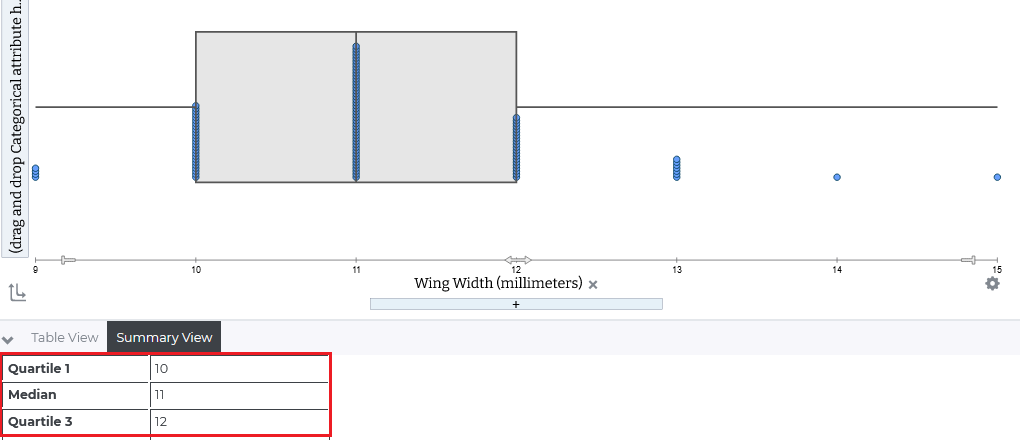# Box Plot Quartiles in Tuva

When an array of numbers or data values is sorted in ascending manner and divided into four roughly equal parts, the subsets are called quartiles. The upper quartile is the part containing the highest data values, the upper-middle quartile is the part containing the next-highest data values, the lower middle quartile is the part containing the next-lowest data values while the lower quartile is the part containing the lowest data values.

The term quartile can have two connotations:

• It can refer to the subset of all data values in each of those parts.
• It can refer to cut-off values between the subsets (Q1, Q2, and Q3).

In the 5-number summary, it refers to the cut-off values between the subsets.

Statisticians disagree on whether the quartile values should be actual points from the data set itself, or whether they can fall between the points (as the median can when there are an even number of data points). Further, most data sets don't have a unique set of values (Q1, Q2, Q3) that divides the data into four roughly equal parts. As a result, we have several methods for computing the quartiles and the choice largely depends on the objective of the investigation.

Note:

1. The Tuva Tools allows students to work with large datasets with facility. For large datasets, differences among algorithms used for computing quartiles become less noticeable and are often unimportant.
2. In Tuva we focus on reducing the procedural drudgery of manually computing quartiles for large datasets and focusing on interpreting what the quartiles tell you about the data. Learning to manually compute quartiles has its value but once you have learned the underlying method, you no longer need to revisit it every time you are analyzing data.
3. Tuva adopted a method for computing quartiles that has a wider application in computing different quantiles.

## The Interpolation Method

Tuva uses the Interpolation method to find quartiles, with a formula (n-1)*p to locate the quartiles in an array, where n = the number of data values and p= the desired percentile.

If the desired quartile falls between two of the values in the array, Tuva interpolates between these values to calculate the quartile value.

This method yields quartile values equivalent to MS Excel’s inclusive method and it finds a wider application in finding different types of quantiles such as quintiles and percentiles.

Here’s an example dataset: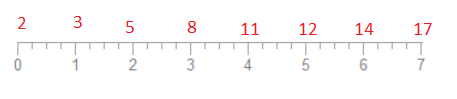2, 3, 5, 8, 11, 12, 14, 17 (n=8) (https://tuvalabs.com/upload/d/ba967898dfa54d8b8416f4c9aed474e1/)

The values are laid out on the integer positions of the number line: starting at zero and ending with N-1. The N values are distributed over a length of  N-1.

Q1 index = (8-1)*0.25 = 1.75

Q2 index = (8-1)*0.5 = 3.5

Q3 index = (8-1)*0.75 = 5.25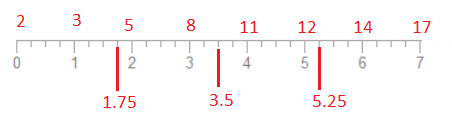The data value corresponding to each index is found by interpolation.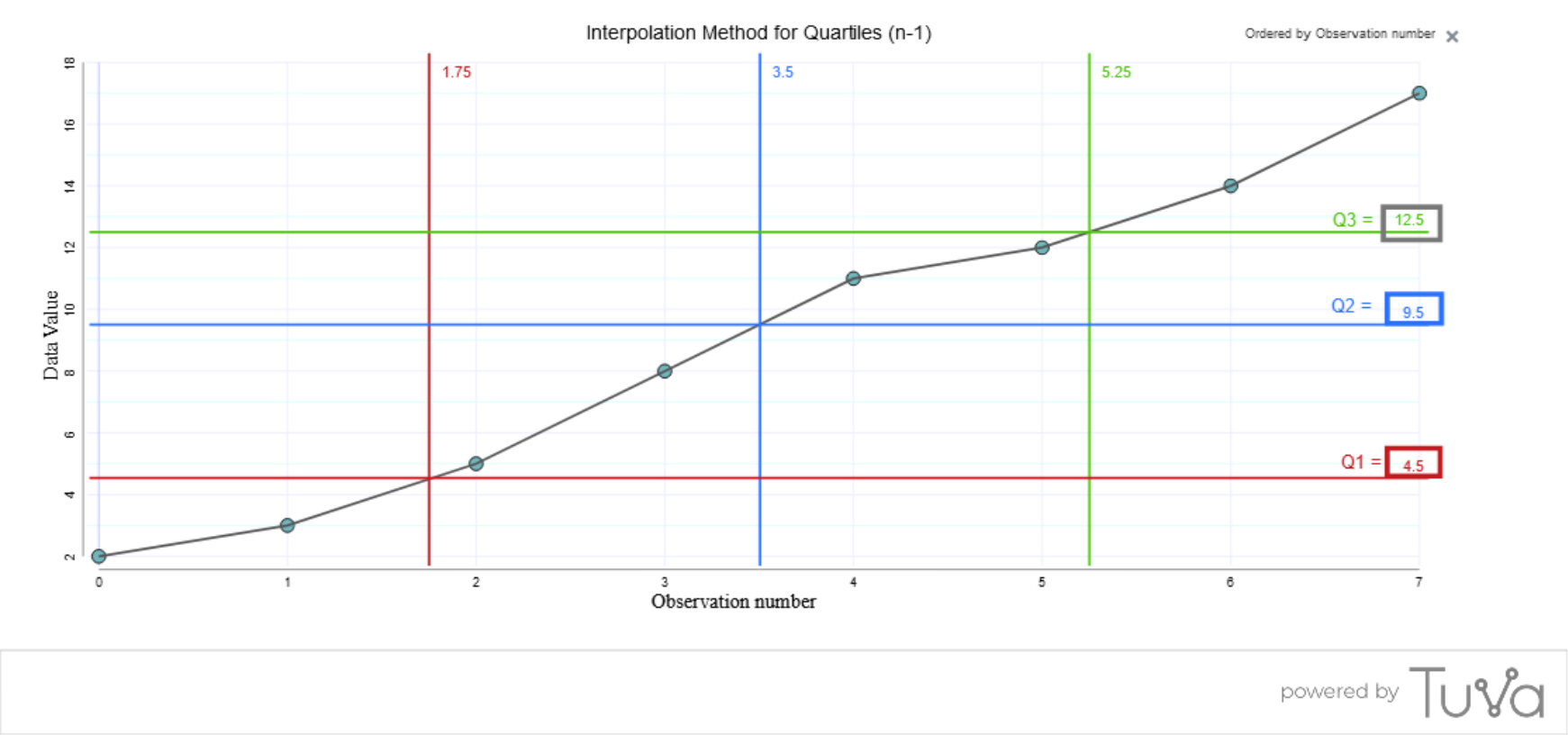The actual box plot looks like this: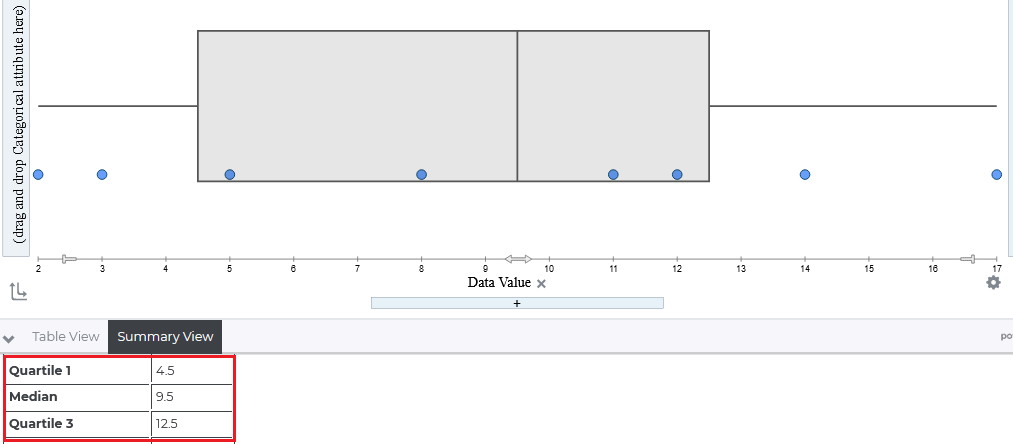Here’s an example with the Cicadas dataset: